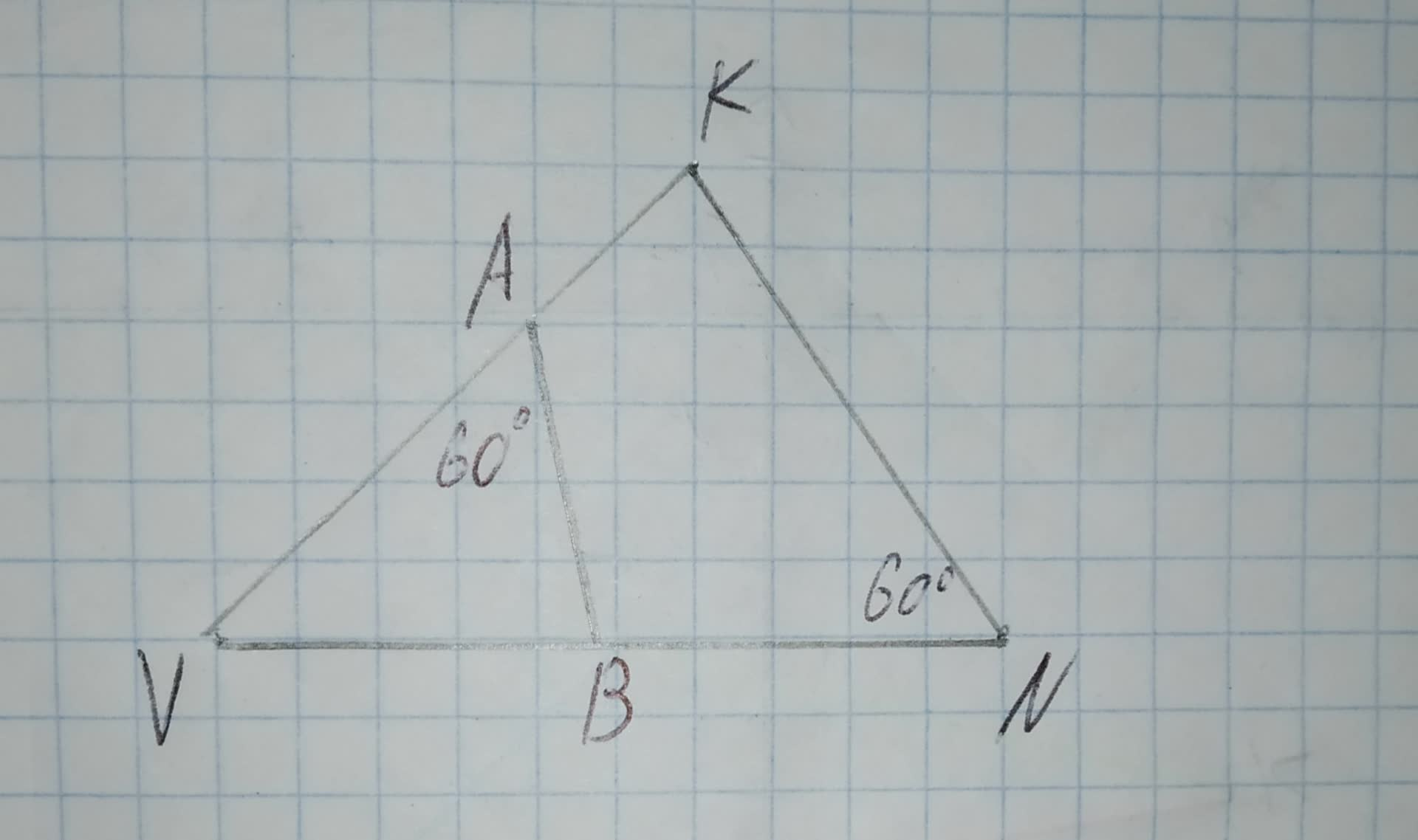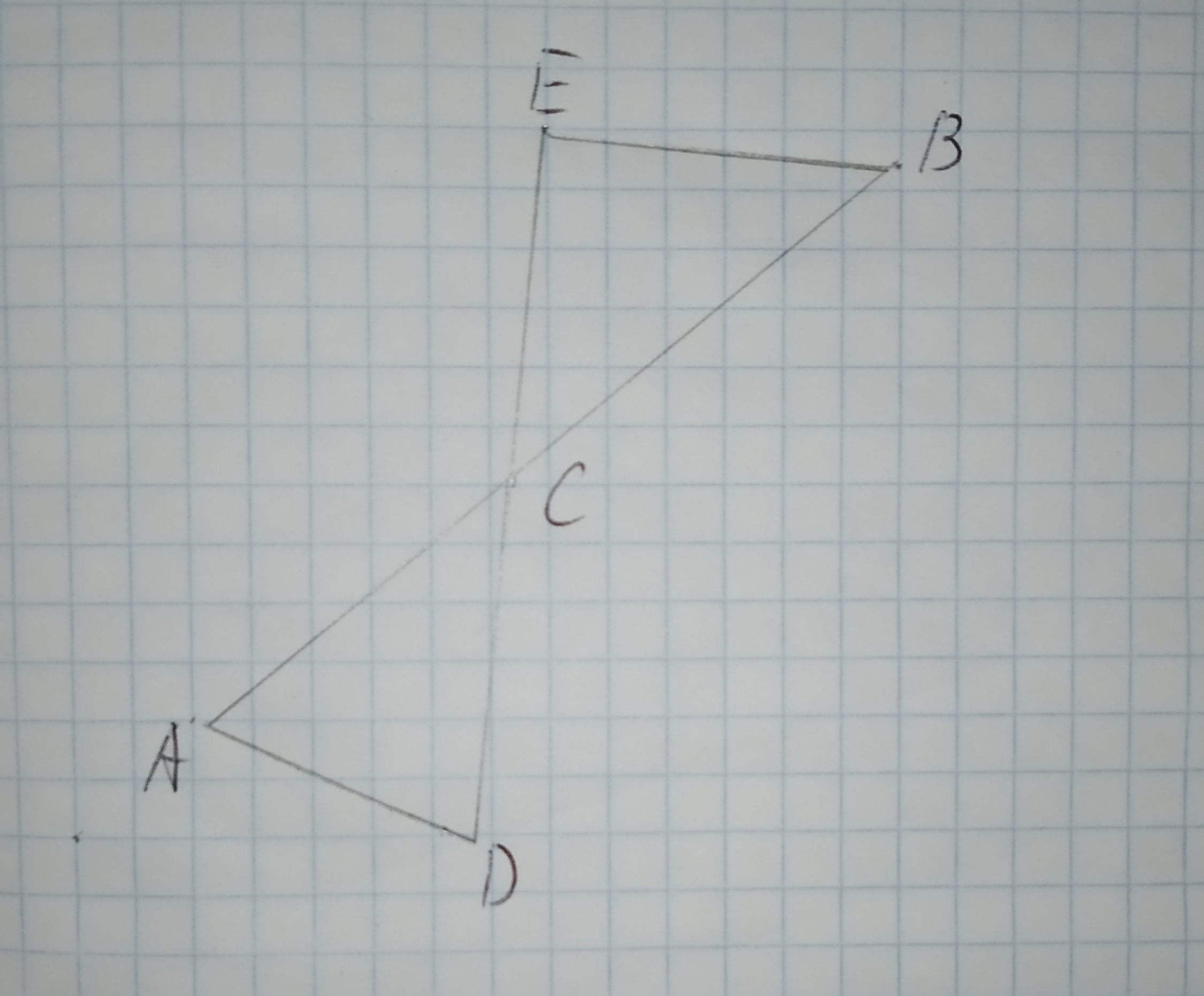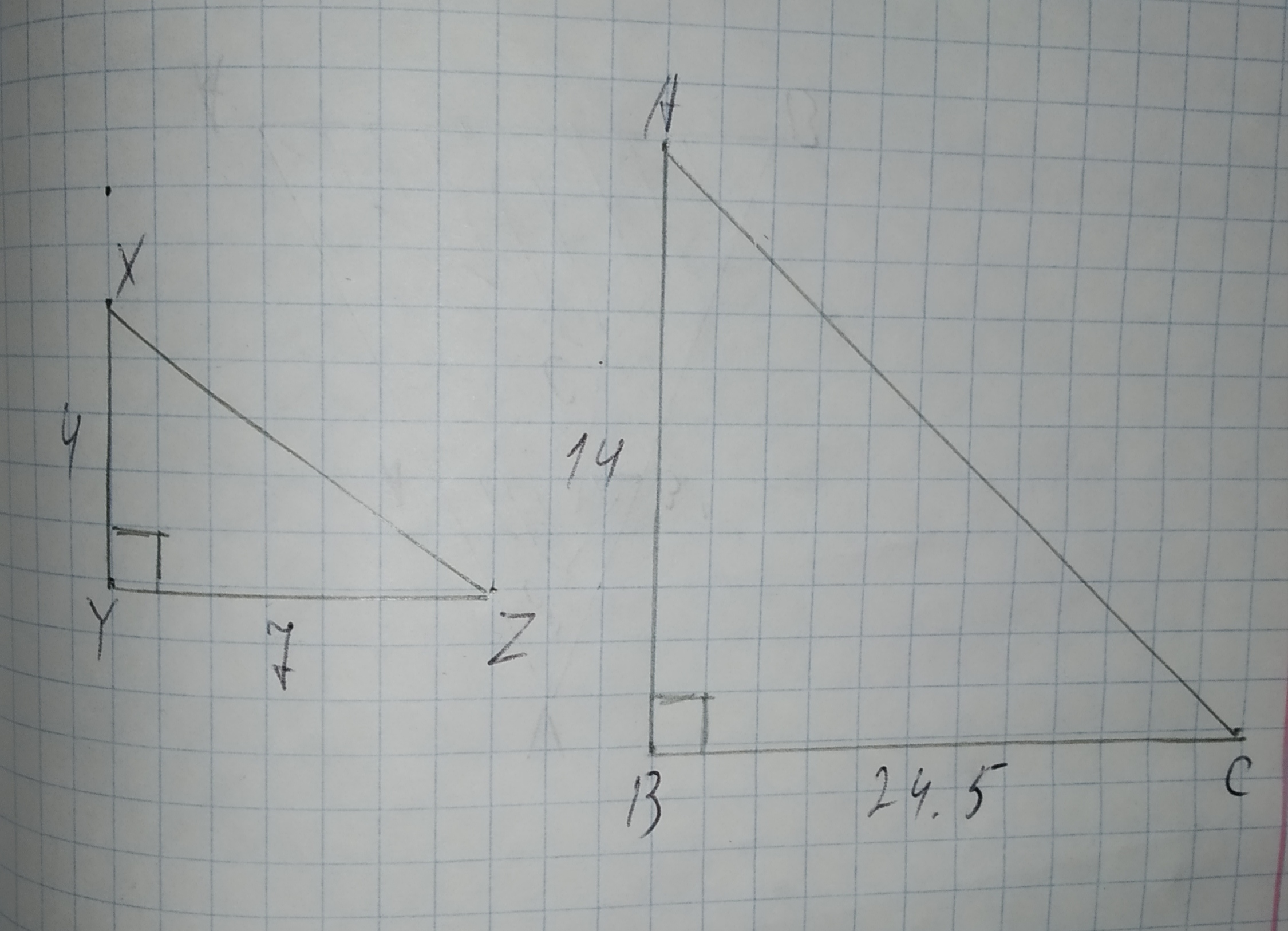# To determine:To prove:The congruency of /_PBC ~=/_PBD. Given information: The following information has been given /_BPC=/_BPD=90^(circ) /_C=/_DQuestion
SimilarityTo determine:To prove:The congruency of $$\displaystyle\angle{P}{B}{C}\stackrel{\sim}{=}\angle{P}{B}{D}$$.
Given information:
The following information has been given
$$\displaystyle\angle{B}{P}{C}=\angle{B}{P}{D}={90}^{{\circ}}$$
$$\displaystyle\angle{C}=\angle{D}$$2020-12-30
Formula used : If any two triangles have two common angles, then by virtue of similarity, third angle will also be congruent
Proof : In triangles PBC and PBD, we have
$$\displaystyle\angle{B}{P}{C}=\angle{B}{P}{D}={90}^{{\circ}}$$
$$\displaystyle\angle{C}=\angle{D}$$
Thus, by virtue of similarity, third angle will also be congruent. Hence,
$$\displaystyle\angle{P}{B}{C}\stackrel{\sim}{=}\angle{P}{B}{D}$$
Thus, the given congruency is proved

### Relevant QuestionsTo prove:The congruency of $$\displaystyle\vec{{{M}{H}}}\stackrel{\sim}{=}\vec{{{J}{O}}}$$.
Given information:
The following information has been given GJKM is a rhombus
$$\displaystyle\angle{J}{O}{G}=\angle{M}{H}{G}={90}^{{\circ}}$$To prove: The congruency of $$\displaystyle\triangle{R}{K}{M}\stackrel{\sim}{=}\triangle{O}{M}{K}$$.
Given information: The following information has been given
$$\displaystyle\angle{M}{R}{K}=\angle{K}{O}{M}={90}^{{\circ}}$$
$$\displaystyle\angle{R}{K}{M}=\angle{O}{M}{K}$$
KM is the common chordTo prove:The congruency of $$\displaystyle\vec{{{S}{O}}}\stackrel{\sim}{=}\vec{{{T}{O}}}$$.
Given information: The following information has been given O is the center of the circle
$$\displaystyle\angle{S}{O}{V}=\angle{T}{O}{W}$$
$$\displaystyle\angle{W}{S}{O}=\angle{V}{T}{O}$$To determine: The value of segment HF
Given information:
The following information has been given
GF=6
EG=9To determine: The value of segment HF
Given information:
The following information has been given
EH=7
HG=4To determine: The value of segment HF
Given information:
The following information has been given
EH=7
HG=3Determine whether $$\displaystyle\triangle{A}{B}{C}{\quad\text{and}\quad}\triangle{D}{E}{F}$$ are similar for each set of measures. If so, identify the similarity criterion.
1.$$\displaystyle{m}\angle{A}={20},{m}\angle{C}={20},{m}\angle{D}={40},{m}\angle{F}={40}$$
2.$$\displaystyle{m}\angle{A}={20},{m}\angle{C}={4}{u},{m}\angle{D}={20},{m}\angle{F}={40}$$
3.$$\displaystyle{A}{B}={20},{B}{C}={40},{m}\angle{B}={53}$$,
$$\displaystyle{D}{E}={10},{E}{F}={20},{m}\angle{E}={53}$$To write: the name of the postulate, that justifies the given statement.
Given information:In $$\displaystyle\triangle{A}{V}{B}{\quad\text{and}\quad}\triangle{N}{V}{K},\angle{A}=\angle{N}={60}^{{\circ}}$$.
The given figure is as follows:To check: whether the additional information in the given option would be enough to prove the given similarity.
Given:
The given similarity is $$\displaystyle\triangle{A}{D}{C}\sim\triangle{B}{E}{C}$$The given options are:
A.$$\displaystyle\angle{D}{A}{C}{\quad\text{and}\quad}\angle{E}{C}{B}$$ are congruent.
B.$$\displaystyle\overline{{{A}{C}}}{\quad\text{and}\quad}\overline{{{B}{C}}}$$ are congruent.
C.$$\displaystyle\overline{{{A}{D}}}{\quad\text{and}\quad}\overline{{{E}{B}}}$$ are parallel.
D.$$\displaystyle\angle{C}{E}{B}$$ is a right triangle.